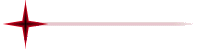##Astronomical Units

Last revised: 05/22/01 07:28 PM

### Some common units and conversions:

 Unit Symbol Value/Conversion Unit Astronomical Unit (the average distance from the center of the Earth to the center of the Sun) AU or  ua 1.496 x 1013 cm 1.496 x 1011 m 1.496 x 108 km 9.296 x 107 mi Light Year (distance that light travels in one year) ly 9.46 x 1017 cm 9.46 x 1015 m 9.46 x 1012 km 5.88 x 1012 mi 63,253 AU Parsec (distance at which a star appears to shift its position by one arcsecond over the  time [about 3 months] in which the Earth moves a distance of one AU in the direction perpendicular to the direction to the star) pc 648,000/pi AU (by definition) 3.09 x 1018 cm 3.09 x 1016 m 3.09 x 1013 km 1.92 x 1013 mi 206,264.8 AU 3.261 ly hubble hubble 109 ly (by definition) 306.59 Mpc Velocity of light (a unit of velocity equal to the speed of light in a vacuum) c 2.998 x 1010 cm/s 2.998 x 108 m/s 299,792.458 km/s 1.079 x 109 km/hr 186,282.583 mi/s 6.707 x 108 mi/hr Hubble constant H0 60..75 km/s/Mpc 18..23 km/s/ly Earth Gravitational constant (average acceleration produced by gravity at the Earth's surface) g 9.807 m/s2 32.174 ft/s2 Earth radius Re 6.378 x 106 m 6,378 km 3,986 mi Solar radius Rs 6.96 x 108 m 696,000 km 435,000 mi Earth Mass Me 5.98 x 1024 kg 1.318 6 x 1028 lb Solar Mass Ms 1.99 x 1030 kg 4288 x 1033 lb Kilometer km 1000 m 0.6214 mi Miles mi 1.609 km Ton t (US short) 2000 lb megaton of TNT Mt 4.1 x 1022 ergs 4.1 x 1015 joules 4.4 x 1014 kg-meters 1.13 x 1012 kilowatt-hrs 3.06 x 1012 Btu Ergs .0000001 joules 1.02 x 10-8 kg-meters 2.78 x 10-11 kilowatt-hrs 7.48 x 10-11 Btu

### Exponent & Name Chart:

 Number Name Power of Ten One 10^0 Ten 10^1 Hundred 10^2 Thousand 10^3 Million or mega 10^6 Billion 10^9 Trillion 10^12 Quadrillion 10^15 Quintillion 10^18 Sextillion 10^21 Septillion 10^24 Octillion 10^27 Nonillion 10^30 Decillion 10^33 Undecillion 10^36 Dodecillion 10^39 Tredecillion 10^42 Quattuordecillion 10^45 Quindecillion 10^48 Sexdecillion 10^51 Septendecillion 10^54 Octodecillion 10^57 Novemdecillion 10^60 Vigintillion 10^63 Unvigintillion 10^66 Dovigintillion 10^69 Trevigintillion 10^72 Quattuorvigintillion 10^75 Quinvigintillion 10^78 Sexvigintillion 10^81 Septenvigintillion 10^84 Octovigintillion 10^87 Novemvigintillion 10^90 Trigintillion 10^93 Unrtigintillion 10^96 Dotrigintillion 10^99 Googol 10^100 Tretrigintillion 10^102 Quattuortrigintillion 10^105 Quintrigintillion 10^108 Sextrigintillion 10^111 Septentrigintillion 10^114 Octotrigintillion 10^117 Novemtrigintillion 10^120 Googolplex 10^10googols

There is no rational need for any larger number to exist in this universe because there are no more than a Trigintillion (10^93) elemental particles in the known universe.  There aren't even enough particles in the universe to write the number!